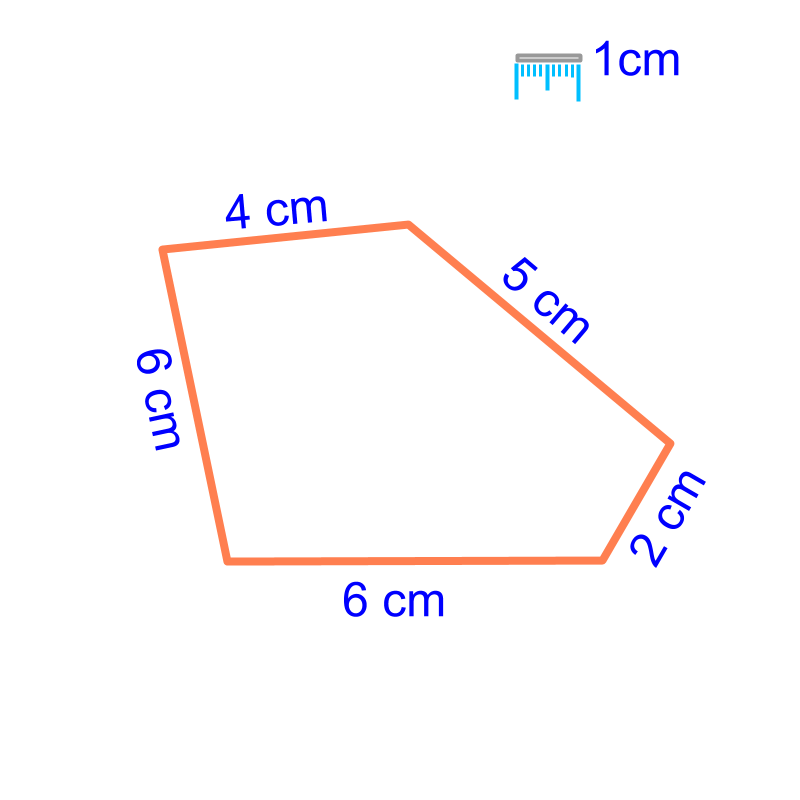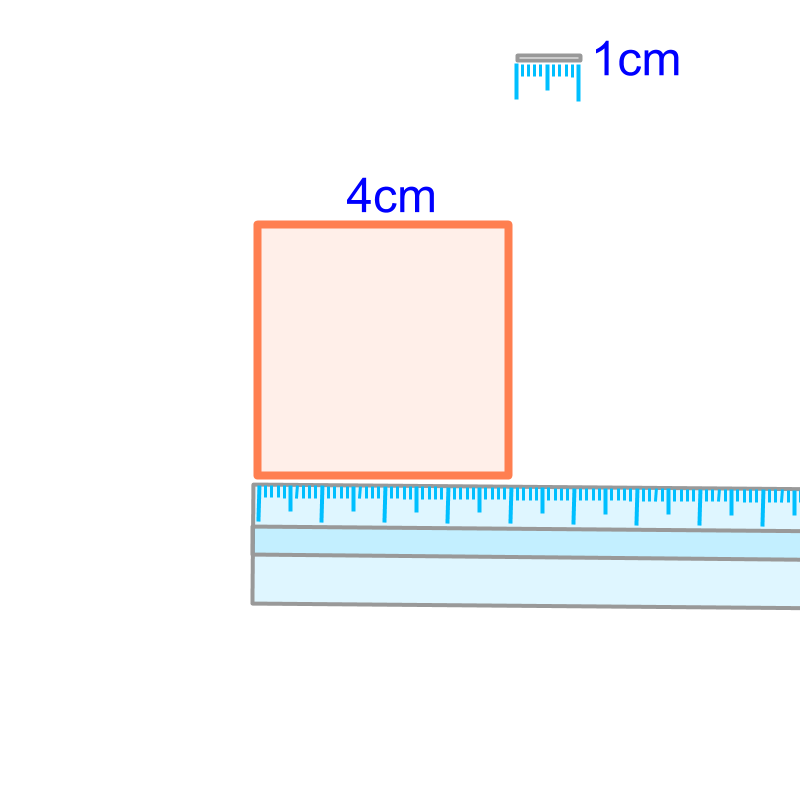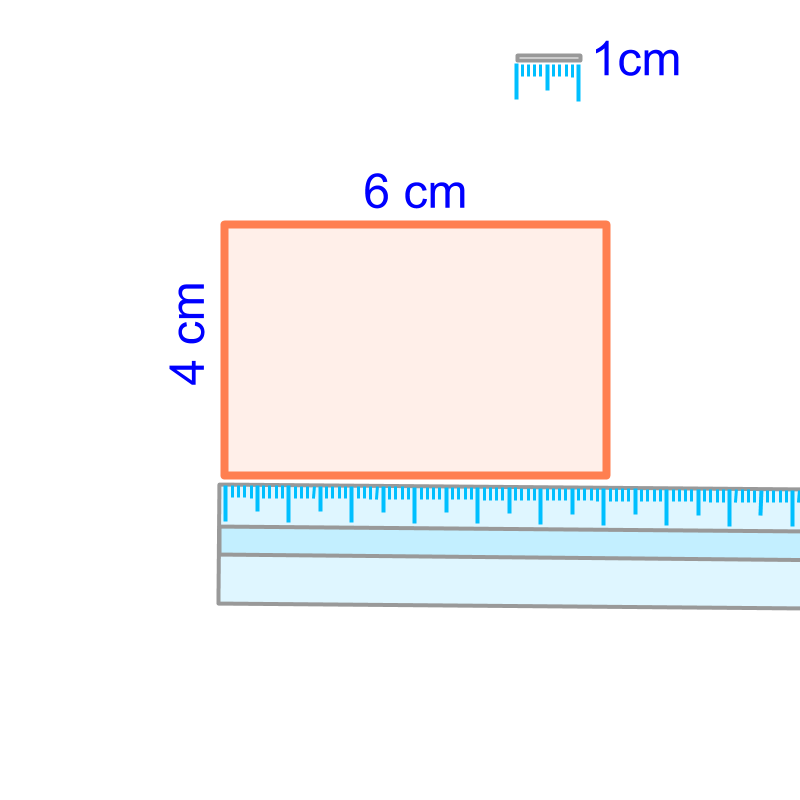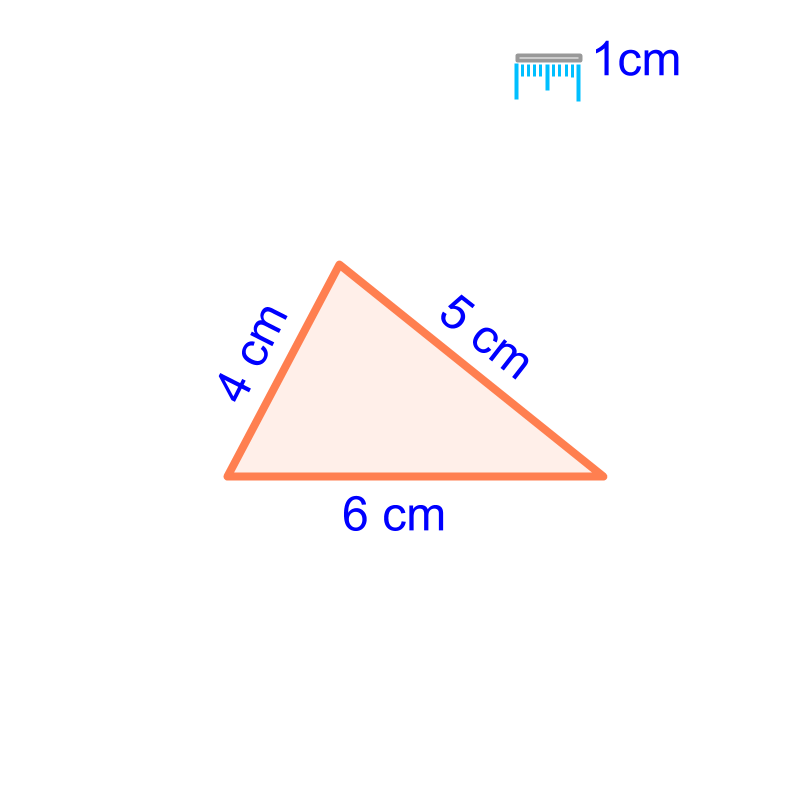maths > mensuration-basics

Perimeter: Square, Rectangle, Triangle, Polygons

what you'll learn...

Overview

Perimeter :
Perimeter of a figure is the length of the line or curve forming the boundary of the figure.

Perimeter of square $=4×\phantom{\rule{1ex}{0ex}}\text{side}$$= 4 \times \textrm{s i \mathrm{de}}$

Perimeter of rectangle $=2×\phantom{\rule{1ex}{0ex}}\text{length + width}$$= 2 \times \textrm{\le n > h + w i \mathrm{dt} h}$

Perimeter of triangle $=\phantom{\rule{1ex}{0ex}}\text{sum of three sides}$$= \textrm{\sum o f t h r e e s i \mathrm{de} s}$

Perimeter of polygon $=\phantom{\rule{1ex}{0ex}}\text{sum of sides}$$= \textrm{\sum o f s i \mathrm{de} s}$perimeter

The measure of length is the "distance-span between two points".

Perimeter of a figure is the length of the line or curve forming the boundary of the figure.

The word "perimeter" means: measure around a shape.

Perimeter is from the Greek root words peri (around) and meter (measure).The perimeter of a square of side $3$$3$ cm is $3+3+3+3=4×3=12$$3 + 3 + 3 + 3 = 4 \times 3 = 12$ cm.

Perimeter of a square is $4$$4$ times the length of the side.The perimeter of a rectangle of length $6$$6$ cm and width $4$$4$ cm is $6+4+6+4=2×\left(6+4\right)=20$$6 + 4 + 6 + 4 = 2 \times \left(6 + 4\right) = 20$ cm.

Perimeter of a rectangle is twice the sum of length and width.The perimeter of a triangle of sides $6$$6$ cm, $5$$5$ cm, and $4$$4$ cm is $6+5+4=15$$6 + 5 + 4 = 15$ cm.

The perimeter of a triangle is sum of the lengths of the three sides.The perimeter of a polygon of sides, $6$$6$ cm, $2$$2$ cm, $5$$5$ cm, $4$$4$ cm, and $6$$6$ cm is $6+2+5+4+6=23$$6 + 2 + 5 + 4 + 6 = 23$ cm.

Perimeter of a polygon is the sum of the lengths of its sides.

examples

What is the perimeter of a equilateral triangle with sides, $1$$1$cm?
The answer is "$1+1+1=3$$1 + 1 + 1 = 3$ centimeter".

What is the perimeter of a rectangle formed by joining $2$$2$ squares of side $3$$3$ cm?
The answer is "$18$$18$cm".

Two squares are joined and the rectangle has length $3+3=6$$3 + 3 = 6$cm and height $3$$3$cm.
So the perimeter is $2\left(6+3\right)=18$$2 \left(6 + 3\right) = 18$ cm.

What is the perimeter of regular pentagon of side $2$$2$cm?
The answer is "$10$$10$cm".

summary

Perimeter :
Perimeter of a figure is the length of the line or curve forming the boundary of the figure.

Perimeter of square $=4×\phantom{\rule{1ex}{0ex}}\text{side}$$= 4 \times \textrm{s i \mathrm{de}}$

Perimeter of rectangle $=2×\phantom{\rule{1ex}{0ex}}\text{length + width}$$= 2 \times \textrm{\le n > h + w i \mathrm{dt} h}$

Perimeter of triangle $=\phantom{\rule{1ex}{0ex}}\text{sum of three sides}$$= \textrm{\sum o f t h r e e s i \mathrm{de} s}$

Perimeter of polygon $=\phantom{\rule{1ex}{0ex}}\text{sum of sides}$$= \textrm{\sum o f s i \mathrm{de} s}$Outline

The outline of material to learn "Mensuration basics : Length, Area, & Volume" is as follows.

•  Measuring Basics

→   Introduction to Standards

→   Measuring Length

→   Accurate & Approximate Meaures

→   Measuring Area

→   Measuring Volume

→   Conversion between Units of Measure

•  2D shapes

→   Perimeter of Polygons

→   Area of Square & rectangle

→   Area of Triangle

→   Area of Polygons

→   Perimeter and area of a Circle

→   Perimeter & Area of Quadrilaterals

•  3D shapes

→   Surface Area of Cube, Cuboid, Cylinder

→   Volume of Cube, Cuboid, Cylinder